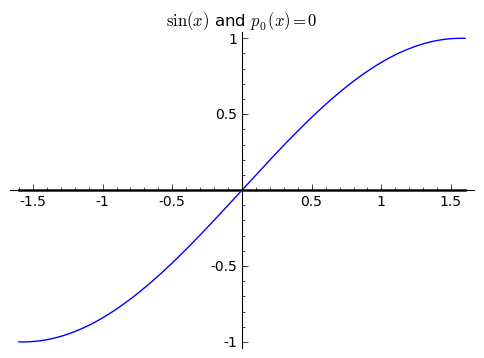# Tag Archives: taylor polynomials

## An Intuitive Overview of Taylor Series

This is a note written for my fall 2013 Math 100 class, but it was not written “for the exam,” nor does anything on here subtly hint at anything on any exam. But I hope that this will be helpful for anyone who wants to get a basic understanding of Taylor series. What I want to do is try to get some sort of intuitive grasp on Taylor series as approximations of functions. By intuitive, I mean intuitive to those with a good grasp of functions, the basics of a first semester of calculus (derivatives, integrals, the mean value theorem, and the fundamental theorem of calculus) – so it’s a mathematical intuition. In this way, this post is a sort of follow-up of my earlier note, An Intuitive Introduction to Calculus.

PLEASE NOTE that my math compiler and my markdown compiler sometimes compete, and sometimes repeated derivatives are too high or too low by one pixel.

We care about Taylor series because they allow us to approximate other functions in predictable ways. Sometimes, these approximations can be made to be very, very, very accurate without requiring too much computing power. You might have heard that computers/calculators routinely use Taylor series to calculate things like ${e^x}$ (which is more or less often true). But up to this point in most students’ mathematical development, most mathematics has been clean and perfect; everything has been exact algorithms yielding exact answers for years and years. This is simply not the way of the world.

Here’s a fundamental fact to both mathematics and life: almost anything worth doing is probably pretty hard and pretty messy.

For a very recognizable example, let’s think about finding zeroes of polynomials. Finding roots of linear polynomials is very easy. If we see ${5 + x = 0}$, we see that ${-5}$ is the zero. Similarly, finding roots of quadratic polynomials is very easy, and many of us have memorized the quadratic formula to this end. Thus ${ax^2 + bx + c = 0}$ has solutions ${x = \frac{-b \pm \sqrt{b^2 – 4ac}}{2a}}$. These are both nice, algorithmic, and exact. But I will guess that the vast majority of those who read this have never seen a “cubic polynomial formula” for finding roots of cubic polynomials (although it does exist, it is horrendously messy – look up Cardano’s formula). There is even an algorithmic way of finding the roots of quartic polynomials. But here’s something amazing: there is no general method for finding the exact roots of 5th degree polynomials (or higher degree).

I don’t mean We haven’t found it yet, but there may be one, or even You’ll have to use one of these myriad ways – I mean it has been shown that there is no general method of finding exact roots of degree 5 or higher polynomials. But we certainly can approximate them arbitrarily well. So even something as simple as finding roots of polynomials, which we’ve been doing since we were in middle school, gets incredibly and unbelievably complicated.

So before we hop into Taylor series directly, I want to get into the mindset of approximating functions with other functions.

1. Approximating functions with other functions

We like working with polynomials because they’re so easy to calculate and manipulate. So sometimes we try to approximate complicated functions with polynomials, a problem sometimes called
polynomial interpolation”.

Suppose we wanted to approximate ${\sin(x)}$. The most naive approximation that we might do is see that ${\sin(0) = 0}$, so we might approximate ${\sin(x)}$ by ${p_0(x) = 0}$. We know that it’s right at least once, and since ${\sin(x)}$ is periodic, it’s going to be right many times. I write ${p_0}$ to indicate that this is a degree ${0}$ polynomial, that is, a constant polynomial. Clearly though, this is a terrible approximation, and we can do better.